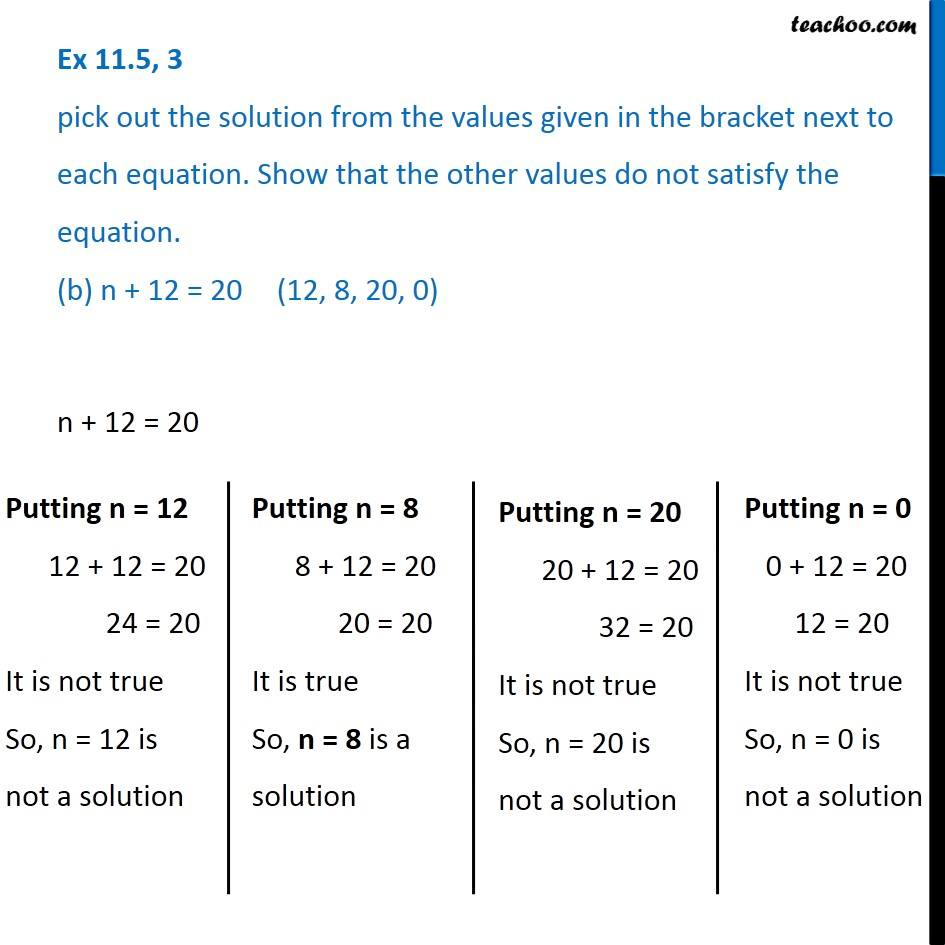Ex 11.5

Chapter 11 Class 6 Algebra
Serial order wise### Transcript

Ex 11.5, 3 pick out the solution from the values given in the bracket next to each equation. Show that the other values do not satisfy the equation. (b) n + 12 = 20 (12, 8, 20, 0)n + 12 = 20 Putting n = 12 12 + 12 = 20 24 = 20 It is not true So, n = 12 is not a solution Putting n = 8 8 + 12 = 20 20 = 20 It is true So, n = 8 is a solution Putting n = 20 20 + 12 = 20 32 = 20 It is not true So, n = 20 is not a solution Putting n = 0 0 + 12 = 20 12 = 20 It is not true So, n = 0 is not a solution